# Annotated Cosmic Call Primer

The Cosmic Call was a radio message sent from a 70-meter radio transmitter in Evpatoria, Ukraine, to four stars in 1999 and five more stars in 2003. It had a number of elements: a primer explaining basic aspects of human math, science, and biology, followed by digitized drawings, texts, videos and songs. This webpage discusses the primer only.

This annotation of the primer is based on explanatory documents written by Dutil and Dumas, with a little bit of consolidation and cleanup by Michael Chorost. A short history of the message’s creation was written by Chorost here. An in-depth discussion of the primer is here.

There are 23 pages in the primer. Each page consists of a box 127 pixels on a side with a one-pixel border around it. The top left and right corners of each page give its page number in binary, e.g. 00001 is page 1, 00010 is page 2, 00011 is page 3, and so forth. The center symbol at the top represents the topic of the page.

The symbols are designed to be resistant to signal degradation. A single flipped bit could make an 8 into a 0, or a 1 into a 7. They are also designed to be hard to confuse with each other even if corrupted by noise. Furthermore, none of the symbols are rotated or mirrored images of any others, so the message will still be intact even if the recipients construct it upside-down or in mirror-reverse.

The message was sent to the following stars.

The Cosmic Call 1999 Target Stars

Name Designation HD Constellation Spectral type Distance Date sent Arrival date Planets?
16 Cyg HD 186408 Cygnus G2V 70.5 May 24, 1999 November 2069 Binary star system. 16 Cygni B has 1 gas giant whose orbit is partly in the habitable zone (HZ).
15 Sge HD 190406 Sagitta G1V 57.6 June 30, 1999 February 2057
HD 178428 Sagitta G5V 68.3 June 30, 1999 October 2067
Gl 777 HD 190360 Cygnus G6IV+ 51.8 July 1, 1999 April 2051 2 planets, neither in the HZ
Sources: Zaitsev, Alexander and Ignatov, Sergey (1999), Report on Cosmic Call 1999, Tables 1 and 2, and Zaitsev, Alexander (2004), “The transfer and the search for intelligent signals in the universe,” Table 3. Planetary data are from Abel Méndez, curator of the Habitable Exoplanets Catalog.

Dutil and Dumas revised the primer for a new set of messages sent in 2003. Their goal was to compress it to save transmission time. They eliminated the page divisions, reduced the pixel size of the numerals, and removed page 14 because they felt it would be more confusing than helpful. Other than that, the 2003 primer is essentially the same as the 1999 one.

The Cosmic Call 2003 Target Stars

Name Designation HD Constellation Spectral type Distance Date sent Arrival date Planets?
Hip 4872 Cassiopeia K5V 32.8 July 6, 2003 April 2036
Gl 208 HD 245409 Orion K7 37.1 July 6, 2003 August 2040
55 Cnc HD 75732 Cancer G8V 40.3 July 6, 2003 May 2044 5 planets, one of which is a gas giant in the HZ.
HD 10307 Andromeda G1.5V 41.5 July 6, 2003 September 2044
47 UMa HD 95128 Ursa Major G1V 45.9 July 6, 2003 May 2049
Sources: Sources for 2003 target stars: Zaitsev, Alexander (2004), “The transfer and the search for intelligent signals in the universe,” Table 3. Planetary data are from Abel Méndez, curator of the Habitable Exoplanets Catalog.

### Page 1. Numbers

 This defines the numbers used in the message. It lists the numbers 0 to 20, reading across and omitting several of them. Each number is given in three forms: as a group of dots, as a binary number, and as a symbol in base-10 format. The symbol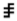means “equals.” So the line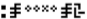translates into 2 = 2 = 2. Below are are the prime numbers 2, 3, 5, 7, 11, 13, 17, 19, 23, 29, 31, 37, 41, 43, 47, 53, 59, 61, 67, 71, 73, 79, 83, and 89. The number at the bottom is the largest known prime in 1999: 23,021,377 – 1. This is given as a clue to our computational abilities.

### Page 2. Operations

This page defines the symbols for addition, subtraction, multiplication, and division. The periodic notation ... is introduced using fractions. The symbol for “undetermined” is defined as  one divided by zero. Negative numbers are introduced by subtracting 1 from 0. The symbol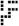means “…”

 1+1=2 1+2=3 3+2=5 4+3=7 1+0=1 1-1=0 1-2=-1 3-2=1 4-3=1 1-0=1 1*1=1 1*2=2 3*2=6 4*3=12 1*0=0 1/1=1 1/2=0.5 3/2=1.5 1/0=undetermined 0/1=0 0-1=-1 1/3=0.3333... 4/3=1.3333... 1/9=0.1111... 2/3=0.6666... 1/11=0.0909...

### Page 3. Exponents

This page demonstrates exponents, which are important for the large numbers that will come in later pages. It uses “ab” notation instead of “a^b” or “a**b” notation to avoid having to cover the mathematical precedence of operations.

 11 =1 12 =1 13 =1 21 =2 22 =4 23 =8 31 =3 32 =9 33 =27 42 =16 53 =125 101 =10 103 =1000 102 =100 10-2 =0.01 1.23*102 =123 45*10-2 =0.45 81/3 =2 41/2 =2 21/2 = 1.4142356...

### Page 4. Variables

This page talks about equations, correspondences, and interrogation. The first line is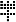X X+2=3  X=1. It can be read as, “What is X? X + 2 = 3.  X=1.”is a variable. At the lower right-hand of the page is a graph with the labels for the X and Y axes.

 ?a ?a ?a ?a ?a ?a ?a a + 2 = 3 a + 4 = 10 a - 5 = 15 a ÷ 2 = 5 a + b = c a * b = c a2 = b a = 1 a = 6 a = 20 a = 10 a = c - b a = c ÷ b a = b0.5 ?a b = a3

### Page 5. Geometry

Geometry is important for discussing length and volume. Pi is presented by showing a circle and its radius. Circumference is distinguished from volume. The first seven digits of pi are given, followed by “...” and the last 15 digits known in 1999.

pi=3.1415927...465698614212904

This is another clue to our computational prowess (although it is possible to calculate the nth digit of pi with the Bailey-Borwein-Plouffe formula.) It can also be used to cross-check the number symbols defined on page 1.

Below that, the Pythagorean theorem is shown.

c2 =a2 +b2
c x c = a x a + b x b

This reinforces the idea of exponents.

### Page 6. Elements

This page introduces the elements. The hydrogen atom is displayed with a proton and electron, along with their respective masses and charges. The proton’s mass is given in relation to the electron’s.

Mass proton = 1836 x Mass electron

Ten elements are enumerated in this page by showing the number of protons and neutrons in their nuclei, using the symbol for union.

 H = 1p U 0n Al = 13p U 14n He = 2p U 2n Si = 14p U 14n C = 6p U 6n Fe = 26p U 30n N = 7p U 7n Na = 11p U 12n O = 8p U 8n Cl = 17p U 18n

### Page 7. Mass

This page discusses mass. The masses of the proton, neutron and electron are given in kilograms, removing the decimal point to make it possible to display more digits. The carbon-12 atom is used to introduce Avogadro’s number, 6.0221367x1023 atoms per mole. Five other elements are introduced for later use in discussing temperature and the composition of the Earth.

 S = 16p U 16n Ag = 47p U 60n Zn = 30p U 35n Au = 79p U 117n Ar = 18p U 22n U = 92p U 116n E112 = 112p U 165n

Element 112 is given to hint at how far humanity has gotten in nuclear physics. (As of 1999, element 113 had not yet been found. Element 114 had been announced but not yet confirmed.)

Mass C = 6 x Mass proton + 6 x Mass neutron + Energy

12 kg = 6022137x1019 x Mass carbon
Mass proton = 16726237 x 10-34 kg
Mass neutron = 16739286 x 10-34 kg
Mass electron = 91093897 x 10-38 kg

### Page 8. The hydrogen atom

This page gives the spectrum of hydrogen, which permits the introduction of symbols for frequency, wavelength, time and energy. At the bottom the speed of light is given using the relationship between its frequency and its wavelength.

 l = 1.87310 x 10 -6 m f = 1.59881 x 10 14 Hz l = 6.56285 x 10 -7 m f = 4.56802 x 10 14 Hz l = 1.21567 x 10 -7 m f = 2.46607 x 10 15 Hz

Second = Hz-1 = 1/Hz
Speed photon = frequency * wavelength = 299792458 m/s

### Page 9. Units of measurement

This page defines units of measurement. The last items are the values of h (Planck's constant) and G (the gravitational constant).

 Force = M x L ÷ T2 1 Newton = 1 kg x m ÷ s2 Energy = M x L2 ÷ T 2 1 Joule = 1 kg x m2 ÷ s2 Pressure = M ÷ L ÷ T2 1 Pascal = 1 kg ÷ m ÷ s2 Power = M x L2 ÷ T3 1 Watt = 1 kg x m2 ÷ s3 Speed = L ÷ T = m ÷ s Acceleration = Speed ÷ T = L ÷ T2 = m ÷ s2 h = 6.6260755 x 10-34 J s G = 6.67259x10-11 m3 kg-1 s-2

### Page 10. Temperatures

The boiling and melting temperatures of some elements are given in Kelvins. The line below these values indicates that these temperatures exist for a pressure of 101300 pascals. At the bottom is a graph showing the freezing and boiling points of water.

 H 14.025 K 20.268 K C 4100 K 4470 K S 388.36 K 717.75 K Zn 692.73 K 1180 K Ag 1234 K 2436 K Au 1337.58 K 3130 K

Pressure = 101300 pascals

HHO 273K 373K

### Page 11. The Solar System

Each planet in our solar system is identified. The masses and radii of Jupiter and the Sun are given to help recipients confirm our solar system as the source of the message. The Sun’s temperature is also given. It is likely that the receiver would be able to measure some of these values independently, so it aids in double-checking comprehension.

Mass Jupiter = 1.901 x 1027 kg

Radius Jupiter = 7.137 x 107 m

Mass Sun = 1.991 x 1030 kg

Radius Sun = 6.9595 x 108 m

Temperature Sun = 5763 K

### Page 12. Earth and Moon (part 1)

This page gives the mass and radius of the Earth and Moon and the distance between them. The last line gives the distance between the Earth and the sun. This will further help in identification of our solar system.

Distance Earth-Moon = 3844 x 105 m
Mass Moon = 7.35 x 1022 kg
Radius Moon = 1.74 x 106 m
Mass Earth = 5.977 x 1024 kg
Radius Earth = 6.378 x 106 m
Distance Earth-Sun = 1.4957 x 1011 m

### Page 13. Earth and Moon (part 2)

This page gives the length of Earth’s day and year, and the duration of the Moon’s orbit around the Earth. It also gives the ages of the Earth and Sun.

Time = 2360591 seconds [Moon orbit]

Time = 31556926 seconds [Earth year]

Time = 1 year

Time = 86163 seconds [Earth day]

Age Earth = 4550000000 years

Age Sun = 4550000000 years

### Page 14. Earth’s crust, water, atmosphere, and surface gravity

This page identifies the elements in Earth’s atmosphere, crust, and oceans. It also notes its surface gravity and its highest and lowest elevations (Mount Everest and the Mariana Trench, respectively, though these are not named in the message.)

 Crust Atmosphere Ocean SiOO AlAlOOO FeFeOOO FeO NN OO Ar COO HHO Na Cl

Top of Land = 8848 m

Bottom of Ocean = 11000 m

Acceleration = 9.7978 m/s2

### Page 15. Exterior human appearance

This page gives a schematic representation of a man and a woman with a mean value of their height in meters. It is adapted from the plaques attached to Pioneer 10 and 11.

The dotted line at the left is a ballistic trajectory intended to clarify which direction is down in a gravitational field.

1.8m height

### Page 16. Other information about humans

This page gives the frequency ranges in which humans hear and see. It also gives our life expectancy, weight and body temperature. The graph on the bottom gives the range of colors perceptible to human vision. It also indicates that we have trichromatic color vision (e.g., our retinas have receptors for wavelengths that we see as blue, green, and red).

People Earth = Male U Female = 6000000000

Age Male = Age Female = 70 years

Mass Male = Mass Female = 80 kg

Temperature Male = Temperature Female = 311 K

Audition: 20Hz to 20,000Hz

Visual: 295 535 565 x10-9m

### Page 17. DNA

This page represents the nucleotides in DNA. Double bonds are omitted. This reveals that we are carbon-based life-forms.

### Page 18. Cells

This page shows a human cell with the DNA in its nucleus. It gives information on cell size and composition, and the number of cells in a human.

6XC U 12xH U 6xO
2xO
C U 2xO

Cell size = 10-5 m
Cell Male = Cell Female = 1013 cells

### Page 19. Map of Earth, left half

This page is the left part of a map representing the Earth’s oceans and continents.

The Fuller projection has been chosen as a way to not center the map on any specific place. Symbols defined on page 14 are used for continents and oceans.

### Page 20. Map of Earth, right half

This page is the right part of a map representing the Earth’s oceans and continents.

### Page 21. The apparatus used to send the message

This page gives information about the radio transmitter used to send the message, and the message itself. The message size is 127x127 pixels by 23 pages. 43,000 people contributed to the message material that came after the primer.

Frequency = 5010240000 Hz
Wavelength = 0.059836 m
127 x 127 x 23
43000 people
Power = 150000 watts
70 meters

At the top left-hand of the page is the “target” symbol (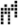). This symbol means “the recipient of the signal” or “you.” This symbol is used on page 23 as a way of directing questions to the recipients.

### Page 22. Cosmology

This page gives some elements of cosmology: the expansion of the universe, the density of the universe, the cosmological constant, the Hubble constant, and the universe’s temperature.

H= 8/3 x π x G x density of the universe + cosmological constant / 3

Density of the universe = 2.76 x 10-27[kg * m-3]

Cosmological constant = 1.08 x 10-35s-2

Hubble constant = 1/4000000000 year-1

Temperature of universe = 2.736 Kelvin

This is also an indication of our technological level and another way to double-check the message.

### Page 23. Questions for Recipients

The purpose of this page is to ask for a reply. It asks questions of the recipients using the symbols established in the previous pages. The large symbol in the center is the one for “variable.” Here, it is the equivalent of a question mark. For example, the first line can be read as, “What is your mass in kilograms?”

The target symbol(defined on the top-left corner of page 21) means “the recipient” or “you.”

? Kilograms
? Meters
? Seconds

? Physics you
? Biology you

? Mass you
? Acceleration you

? Speed
? Acceleration

? Energy
? Force
? Pressure
? Power

? Land you
? Atmosphere you
? Ocean you

? Male
? Female
? People you
? Age you